# NCERT Solutions For Class 10 Maths Chapter 6 Exercise 6.1

## Chapter 6 Ex.6.1 Question 1

Fill in the blanks using the correct word given in brackets:

(i)   All circles are ___________. (congruent, similar)

(ii)  All squares are ___________. (similar, congruent)

(iii) All ___________ triangles are similar. (isosceles, equilateral)

(iv) Two polygons of the same number of sides are similar, if

(a) their corresponding angles are ____________ and

(b) their corresponding sides are ___________. (equal, proportional)

### Solution

(i) All circles are Similar. (congruent, similar)

Reasoning:

As we know that two similar figures have the same shape but not necessarily the same size. (Same size means radii of the circles are equal)

Steps:

Similar. Since the radii of all the circles are not equal.

(ii) All squares are Similar. (similar, congruent)

Reasoning:

As we know that two similar figures have the same shape but not necessarily the same size(same size means sides of the squares are equal.)

Steps:

Similar. Since the sides of the squares are not given equal.

(iii) All Equilateral. triangles are similar. (isosceles, equilateral)

Reasoning:

Anequilateral triangle has equal sides and equal angles.

Steps:

Equilateral. Each angle inan equilateral triangle is $${{60}^{{}^\circ }}$$.

(iv) Two polygons of the same number of sides are similar, if (a) their corresponding angles are Equal and (b) their corresponding sides are Proportional (equal, proportional).

Reasoning:

As we know that two polygons of same number of slides are similar if their corresponding angles are equal and all the corresponding sides are in the same ratio or proportion.

Steps:

(a) Since the polygons have same number of sides, we can find each angle using formula \begin{align}\left( {\frac{{2n - 4}}{n}} \right)\end{align} right angles. Here $$‘n’$$ is number of sides of a polygon.

(b) We can verify by comparing corresponding sides.

## Chapter 6 Ex.6.1 Question 2

Give two different examples of pair of

(i) similar figures

(ii) non-similar figures

### Solution

Steps(i):

(a) Two equilateral triangles of sides $$2$$ cm and $$6$$ cm.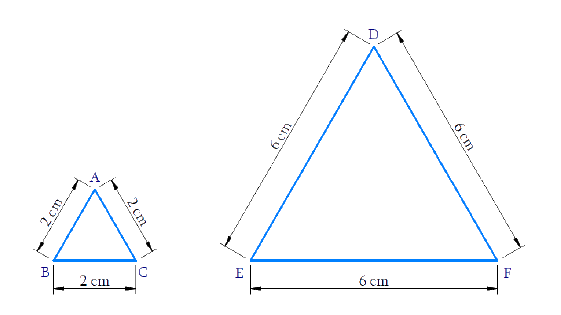$\Delta ABC\sim\Delta DEF\$$\sim\text{is similar to})$ (b) Two squares of sides \(3$$ cm and $$5$$ cm.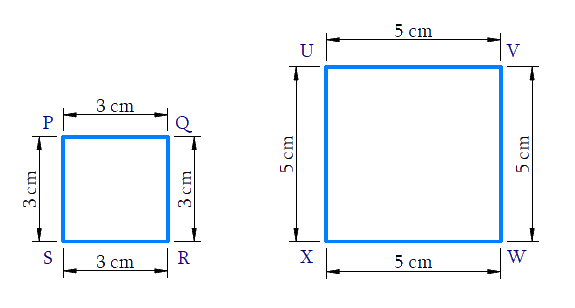$$\square PQRS\!\sim\!\square UVWX\\(\sim\text{is similar to})$$

Steps (ii) :

(a) A quadrilateral and a rectangle.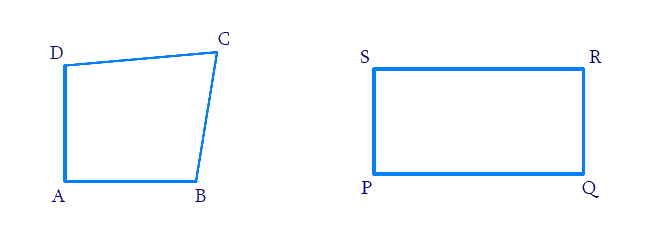(b) A triangle and a parallelogram.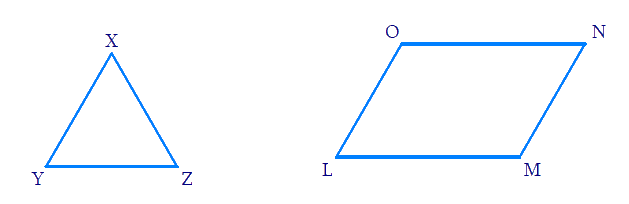## Chapter 6 Ex.6.1 Question 3

State whether the following quadrilaterals are similar or not: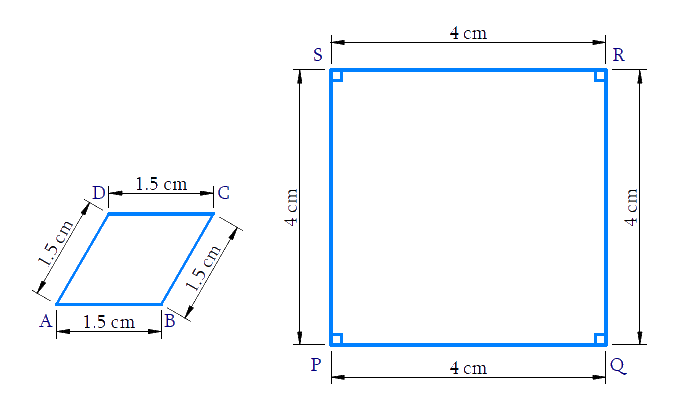### Solution

Reasoning:

Two polygons of the same number of sides are similar, if

(i) all the corresponding angles are equal and

(ii) all the corresponding sides are in the same ratio (or proportion).

Steps:

In Quadrilaterals $$ABCD$$ and $$PQRS$$

\begin{align}\frac{{AB}}{{PQ}} = \frac{{BC}}{{QR}} = \frac{{CD}}{{RS}} = \frac{{DA}}{{SP}} = \frac{{3}}{{8}}\end{align}

$$\Rightarrow$$ Corresponding sides are in proportion

But $$\angle A \ne \angle P;\angle B \ne \angle Q$$

$$\Rightarrow$$ Corresponding angles are not equal

$$\square ABCD \nsim \square PQRS$$

No, Quadrilateral $$ABCD$$ is not similar to Quadrilateral $$PQRS$$

Download SOLVED Practice Questions of NCERT Solutions For Class 10 Maths Chapter 6 Exercise 6.1 for FREE
Ncert Class 10 Exercise 6.1
Ncert Solutions For Class 10 Maths Chapter 6 Exercise 6.1
Instant doubt clearing with Cuemath Advanced Math Program